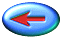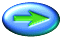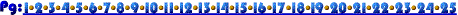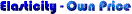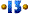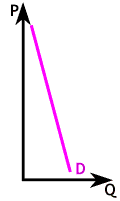Let's examine the graph again. Recall, the arc elasticity demand formula is better in the sense that there is no confusion over the base used, but it's complicated. For now let's compute elasticity simply by using the first price or quantity as the base.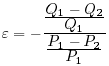The expression to the right is an elasticity formula if we always take the first price and quantity as the base of our percentage computation. It is an easy formula to work with but, as we will see, the elasticity number depends on which number is considered "first."